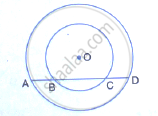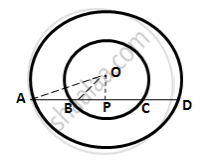Share

The Figure Shows Two Concentric Circles and Ad is a Chord of Larger Circle.Prove That: Ab = Cd - ICSE Class 10 - Mathematics

ConceptChord Properties - the Perpendicular to a Chord from the Center Bisects the Chord (Without Proof)

Question

The figure shows two concentric circles and AD is a chord of larger circle.Prove that: AB = CD

SolutionDrop OP ⊥ AD
∴ OP bisects AD
(Perpendicular drawn from the centre of a circle to a chord bisects it)

⇒ AP = PD      ……………. (i)

Now, BC is a chord for the inner circle and OP ⊥ BC
∴OP bisects BC
(Perpendicular drawn from the centre of a circle to a chord bisects it)
⇒ BP = PC    ……………. (ii)

Subtracting (ii) from (i),
AP - PB = PD - PC
⇒ AB = CD

Is there an error in this question or solution?
Solution The Figure Shows Two Concentric Circles and Ad is a Chord of Larger Circle.Prove That: Ab = Cd Concept: Chord Properties - the Perpendicular to a Chord from the Center Bisects the Chord (Without Proof).
S